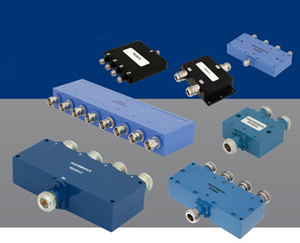# NEWS

## Differences in Power Divider Technology ExplainedA resistive divider entails a relatively simple design, being made up solely of resistors, making them generally inexpensive. A resistive divider incorporates resistors in the paths of the divider. The frequency response of a resistive divider can extend over a wide range of frequencies provided high quality components and proper construction methods are used. A disadvantage of resistive dividers is that the insertion loss is higher: 6 dB for a 2-way power divider as opposed to roughly 3 dB for a reactive or Wilkinson design.

A reactive power divider is a T-junction power divider combined with a quarter-wave transformation. A reactive power divider has a narrower bandwidth than the resistive divider because of the reactive quarter-wave transformer.

A reactive power divider also has lower insertion loss than both resistive and Wilkinson designs and better isolation than a resistive power divider. Reactive power dividers are often used for high power or low passive intermodulation (PIM) applications since they have no resistors.

A Wilkinson power divider has two quarter-wave microstrip lines and utilizes a resistor between the outputs to provide high isolation performance providing the highest isolation of the three power divider designs.

Here are some advantages and disadvantages of the different types of power dividers.Pasternack offers a wide selection of RF power dividers that are in stock for same day shipping.

Latest News: